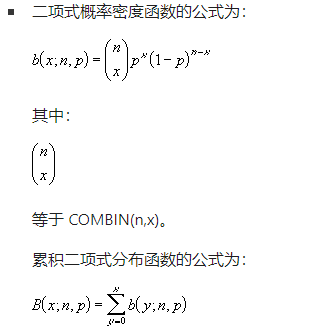### BINOM.DIST函数BINOM.DIST 用于处理固定次数的试验或实验问题。

BINOM.DIST(number_s,trials,probability_s,cumulative)BINOM.DIST 函数语法具有以下参数：

Number_s：必需。 试验的成功次数。

Trials：必需。 独立试验次数。

Probability_s：必需。 每次试验成功的概率。

cumulative：必需。 决定函数形式的逻辑值。

￭ Number_s 和 trials 将被截尾取整。

￭ 如果 number_s、trials 或 probability_s 是非数值型，则 BINOM.DIST 返回 #VALUE! 错误值。

￭ 如果 number_s < 0 或 number_s > trials，则 BINOM.DIST 返回 #NUM! 错误值。

￭ 如果 probability_s < 0 或 probability_s > 1，则 BINOM.DIST 返回 #NUM! 错误值。